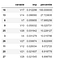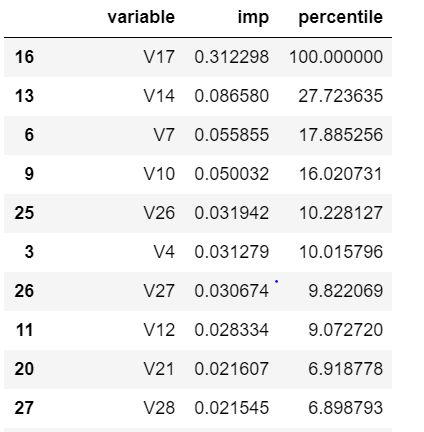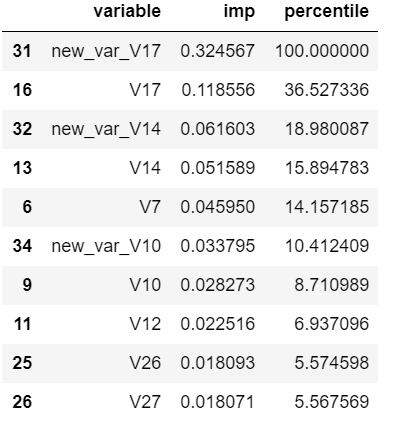# Correlation in XGboost

I have always wondered how robust XGBoost is to correlation among independent variables. Should one check for multicollinearity before building an XGBoost model? In this post I will cover the impact of correlation on XGboost by using two datasets from Kaggle — Credit Fraud Data and BNP Paribas Cardif Claims ManagementPhoto by The Creative Exchange on Unsplash

# Introduction

Correlation is a statistical measure that expresses the extent to which two variables are linearly related (i.e. they change together at a constant rate). It’s a common tool for describing simple relationships without making a statement about cause and effect. The correlation coefficient r measures the strength and direction of a linear relationship, for instance:

• 1 indicates a perfect positive correlation.
• -1 indicates a perfect negative correlation.
• 0 indicates that there is no relationship between the different variables.

There is an ongoing theory that XGBoost output is not effected by the presence of correlated variables in the independent feature set. This is intuitive, since XGBoost is not impacted by the use of one hot encoding for categorical variables. Tianqi Chen has an answer on how XGBoost tackles correlated variables:

This difference has an impact on a corner case in feature importance analysis: the correlated features. Imagine two features perfectly correlated, feature A and feature B. For one specific tree, if the algorithm needs one of them, it will choose randomly (true in both boosting and Random Forests).

However, in Random Forests this random choice will be done for each tree, because each tree is independent from the others. Therefore, approximatively, depending of your parameters, 50% of the trees will choose feature A and the other 50% will choose feature B. So the importance of the information contained in A and B (which is the same, because they are perfectly correlated) is diluted in A and B. So you won’t easily know this information is important to predict what you want to predict! It is even worse when you have 10 correlated features…

In boosting, when a specific link between feature and outcome have been learned by the algorithm, it will try to not refocus on it (in theory it is what happens, the reality is not always that simple). Therefore, all the importance will be on feature A or on feature B (but not both). You will know that one feature has an important role in the link between the observations and the label. It is still up to you to search for the correlated features to the one detected as important if you need to know all of them.

Let us test this theory with some examples and see how XGBoost reacts in case of partially correlated (.8 < r < 1) variables:

# Part 1: Credit fraud data:

The first case uses Credit Card Fraud data from Kaggle. This data has 30 columns — 28 PCA variables, amount, and time. We use the below code snippet to check multicollinear variables in the dataset:

`corr=df.corr()high_corr_var=np.where(corr>0.9)high_corr_var=[(corr.columns[x],corr.columns[y]) for x,y in zip(*high_corr_var) if x!=y and x<y]high_corr_var`

Since the data has PCA variables, the existing feature set is hardly correlated. Hence, for the given analysis we would artificially introduce correlation in the data (details mentioned later).

Prior to that, we first split the data into train and validation (70:30) and estimate the AUC on both datasets (for benchmark):
train-auc:0.997853 , validation-auc:0.98187

Next, we estimate feature importance using below code. Variable V17 comes out to be the most important variable:
`variable_imp=pd.DataFrame({‘variable’:X_train.columns,’imp’:xgb_model.feature_importances_})variable_imp.sort_values(‘imp’,ascending=False,inplace=True)variable_imp[‘percentile’]=variable_imp[‘imp’]*100/variable_imp.iloc[0,1]variable_imp`Top 10 variable based on model importance

Now we artificially introduce fully and partially correlated variables in the model one by one.

Example1: Introducing a perfectly correlated variable with V17 variable .
`X_train[‘new_var’]=3*X_train[‘V17’]+5X_test[‘new_var’]=3*X_test[‘V17’]+5`

Model performance:
train-auc:0.997853 , validation-auc:0.98187Bottom and Top 10 variable based on variable importance

For perfectly correlated variables, there is no impact on model performance — neither on train and nor on validation dataset. Also, there is no change in variable importance and rank order

Example2: Introducing variables partially correlated with top 4 variables of the model:
new_var_v17 corr with v17=0.98
new_var_v14 corr with v14= 0.96
new_var_v7 corr with v7=0.977
new_var_v10 corr with v10=0.973

Model performance :
train-auc:0.998051 , validation-auc: 0.981696Bottom and Top10 variable based on importance

In case of partially correlated features, the output of XGBoost is slightly impacted. We see a marginal change in the performance of the model, suggesting the robustness of XGBoost when dealing with correlated variables.However, one may note that the partially correlated variables in the model are affecting the variable importance.

The above cases talk about a very high correlation between pair of independent variables i.e., in the range of 0.95 to 0.99. The notebook has results of an additional example with a correlation value between 0.85 to 0.90. The same conclusions hold indicating that the XGBoost output is stable irrespective of the value of correlation

# Part2: BNP Paribas Cardif Claims Management

Until now, we have done correlation analysis by adding new correlated variables. Using this data, we will see the impact on performance of XGBoost when we remove highly correlated variables.
The data has 133 variables including both categorical and numerical type. Some pre-processing of data is required — imputing missing variables and label encoding of categorical values.

After the preprocessing, we then split the data into train and validation (70:30) and estimate the AUC on both datasets:
train-auc:0.786068, validation-auc:0.751041

The next step is to remove completely or partially correlated variables from the dataset one at a time and observe the impact on XGBoost output.

Example3:Removing variables having correlation >0.9. A total of 39 variable were removed.
train-auc:0.784501, validation-auc:0.751112

On removing partially correlated variables, we see that there is marginal increase in performance, implying that XGboost is not heavily impacted by removal of the correlated variable.

# Conclusion

This exercise demonstrates that the inclusion of correlated variables affects feature importance for XGBoost model, however the performance of the model remains fairly stable and robust in the presence of multicollinearity.

That’s it for today. Source code can be found on Github. Feel free to share any questions or feedback, connect with me at Linkedin

Reference: